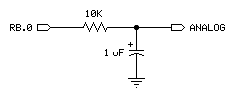PWM Example

PWM Pin, Duty, Duration

Function
Convert a digital value to analog output via pulse-width modulation.

• Pin  is any SX IO pin (RA.0 .. RA.7, RB.0 .. RB.7, RC.0 .. RC.7, RD.0 .. RD.7, RE.0 .. RE.7).
• Duty  is a variable or constant (0 - 255) that specifies the analog output level (0 to 5V).
• Duration  is a variable or constant (0 - 255) that specifies the duration of the PWM output in milliseconds.

Quick Facts
 Units in Duration 1 ms Average voltage equation Average Voltage = (Duty ũ 255) x 5 volts Duration 5 x R x C (suggested) Notes Pin is output while PWM active, then switched to input mode

Explanation
Pulse-width modulation (PWM) allows the SX (a purely digital device) to generate an analog voltage. The basic idea is this: If you make a pin output high, the voltage at that pin will be close to 5V. Output low is close to 0V. What if you switched the pin rapidly between high and low so that it was high half the time and low half the time? The average voltage over time would be halfway between 0 and 5V (2.5V). PWM emits a burst of 1s and 0s with a ratio that is proportional to the duty value you specify.

The proportion of 1s to 0s in PWM is called the duty cycle. The duty cycle controls the analog voltage in a very direct way; the higher the duty cycle the higher the voltage. In the case of the SX, the duty cycle can range from 0 to 255. Duty is literally the proportion of 1s to 0s output by the PWM instruction. To determine the proportional PWM output voltage, use this formula: (Duty ũ 255) x 5V. For example, if Duty is 100, (100 ũ 255) x 5V = 1.96V; PWM outputs a train of pulses to create (through an RC network) an average voltage of 1.96V.

In order to convert PWM into an analog voltage we have to filter out the pulses and store the average voltage. The resistor/capacitor combination shown below will do the job. The capacitor will hold the voltage set by PWM even after the instruction has finished. How long it will hold the voltage depends on how much current is drawn from it by external circuitry, and the internal leakage of the capacitor. In order to hold the voltage relatively steady, a program must periodically repeat the PWM instruction to give the capacitor a fresh charge.

Just as it takes time to discharge a capacitor, it also takes time to charge it in the first place. The PWM command lets you specify the charging time in terms of PWM duration. The timing for the units in Duration is one millisecond.

How do you determine how long to charge a capacitor? Use this rule-of-thumb formula: Charge Time = 5 x R x C. For instance, the circuit below uses a 10 kΩ (10 x 103 ohm) resistor and a 1 ĩF (1 x 10-6 uF) capacitor:Charge time = 5 x 10 x 103 x 1 x 10-6 = 50 x 10-3 seconds, or 50 milliseconds.

```  PWM RB.0, 128, 50                             ' charge to 2.5 volts, 50 ms
```

After outputting the PWM pulses, the SX leaves the pin in input mode (1 in the corresponding bit of the pin's TRIS register). In input mode, the pin's output driver is effectively disconnected. If it were not, the steady output state of the pin would change the voltage on the capacitor and undo the voltage setting established by PWM. Keep in mind that leakage currents of up to 1 ĩA can flow into or out of this "disconnected" pin. Over time, these small currents will cause the voltage on the capacitor to drift. The same applies for leakage current from an op-amp's input, as well as the capacitor's own internal leakage. Executing PWM occasionally will reset the capacitor voltage to the intended value.

PWM charges the capacitor; the load presented by your circuit discharges it. How long the charge lasts (and therefore how often your program should repeat the PWM instruction to refresh the charge) depends on how much current the circuit draws, and how stable the voltage must be. You may need to buffer PWM RC circuit output with a simple op-amp follower if your load or stability requirements are more than the passive circuit can handle.